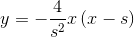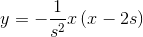KittenYangOK，有了这个思路，我一开始就很直接地如下这么做了：

``````   #define SCROLLDISTANCE  200.0 //滑动的最大距离
...

CGFloat factorOfAngle = 0.0f;
CGFloat Y = MIN(SCROLLDISTANCE,MAX(0,ABS(transition.y)));
if(pan.state == UIGestureRecognizerStateChanged){

factorOfAngle = Y<= SCROLLDISTANCE ? MIN(1,Y / SCROLLDISTANCE) : MAX(0, 1 - MAX(0, (Y - SCROLLDISTANCE) / SCROLLDISTANCE));
CATransform3D t = CATransform3DIdentity;
t.m34  = 1.0/-1000;
t = CATransform3DRotate(t,factorOfAngle*(M_PI/5), transition.y>0?-1:1, 0, 0);
currentPhoto.layer.transform = t;
}

...
````````````     CGFloat Y =MIN(SCROLLDISTANCE,MAX(0,ABS(transition.y)));
factorOfAngle = MAX(0,-4/(SCROLLDISTANCE*SCROLLDISTANCE)*Y*(Y-SCROLLDISTANCE));
``````

``````        CATransform3D t = CATransform3DIdentity;
t.m34  = 1.0/-1000;
t = CATransform3DRotate(t,factorOfAngle*(M_PI/5), transition.y>0?-1:1, 0, 0); //1
``````

//1 最大绕X轴翻转角度为36°，也就是 `M_PI/5```````    ...
factorOfScale = MAX(0,-1/(SCROLLDISTANCE*SCROLLDISTANCE)*Y*(Y-2*SCROLLDISTANCE));
...
t = CATransform3DScale(t, 1-factorOfScale*0.2, 1-factorOfScale*0.2, 0);
``````

//1 当factorOfScale ＝＝ 1时，达到最大缩放状态，为原来的0.8倍（1-0.2）

``````        CGFloat factorOfAngle = 0.0f;
CGFloat factorOfScale = 0.0f;

CGFloat Y =MIN(SCROLLDISTANCE,MAX(0,ABS(transition.y)));

//一个开口向下,顶点(SCROLLDISTANCE/2,1),过(0,0),(SCROLLDISTANCE,0)的二次函数
factorOfAngle = MAX(0,-4/(SCROLLDISTANCE*SCROLLDISTANCE)*Y*(Y-SCROLLDISTANCE));
//一个开口向下,顶点(SCROLLDISTANCE,1),过(0,0),(2*SCROLLDISTANCE,0)的二次函数
factorOfScale = MAX(0,-1/(SCROLLDISTANCE*SCROLLDISTANCE)*Y*(Y-2*SCROLLDISTANCE));

CATransform3D t = CATransform3DIdentity;
t.m34  = 1.0/-1000;
t = CATransform3DRotate(t,factorOfAngle*(M_PI/5), transition.y>0?-1:1, 0, 0);
t = CATransform3DScale(t, 1-factorOfScale*0.2, 1-factorOfScale*0.2, 0);

currentPhoto.layer.transform = t;
``````

PS:这个动画出现在我最近写的一个图片浏览库 `KYElegantPhotoGallery` 中，你可以到这里查看这个优雅的图片展示控件。